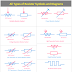The resistor is a very well-known and useful passive component. Resistors are used in almost all electrical and electronic circuits. The main function of a resistor is to block or oppose the flow of electrical current. Another function of the resistor is to convert electrical energy into heat energy. There are various types of resistor are available. So, in this article, we are going to see all types of resistor symbols.

The most common types of resistor are,
• Normal Resistor
• Fixed Resistor
• Variable Resistor
• Preset Resistor or Trimmer Resistor
• Non Reactive Resistor
• Protective Resistor
• Shunt Resistor
• Photoresistor
• Thermal Resistor
• Voltage-Dependent Resistor
• Magneto Resistor
• Iron-Hydrogen Resistor

## Normal Resistor Symbol - IEEE/IEC Standard

Before going to know other types, you must know the symbol of a normal resistor in both IEEE and IEC Standard. IEEE standard symbols are old, they are used in old drawings, designs, and circuit diagrams. IEC standard symbols are new, they are used in modern drawing, diagrams, etc. Anyway, you must know these both types.

## Fixed Resistor Symbol

Here, you can see the symbol of fixed resistor in both IEEE and IEC Standard. It is the most common type of resistor. Fixed Resistor means, its value cannot be varied or changed. The resistance value of the fixed resistor is always constant. Of course, due to the temperature variation, the value may be changed but it is undesirable.

## Variable Resistor Symbol

Variable resistors are those whose resistance values can be varied or changed at any time. The potentiometer is an example of a variable resistor. The symbol of variable resistor is almost the same as the normal resistor. Just the difference is, it is included with an arrow mark that indicates, it is variable.

## Symbol of Preset Resistor

The preset resistor also provides the function to vary its resistance value but we cannot vary the resistance value frequently. Only, during the manufacturing or designing of electronic circuits, its value varied and set to a fixed value. In normal operation, it is not recommended to change its value. But, some preset resistors are designed to vary the resistance value by a screwdriver.

## Non Reactive Resistor Symbol

Non Reactive Resistors are also known as a non-inductive resistors. A normal winded resistor has some inductive reactance, which means it cannot provide a pure resistance value. But non-reactive resistance is designed in such a way that it can eliminate the inductive reactance by producing a counter magnetic field.

## Symbol of Protective Resistor

The protective resistor works like a fuse. It allows the flow of current up to a certain limit. When the flow of current exceeds the limit, the resistor will be blown. Once the protective resistor is blown, it cannot be used again, it should be replaced with a new resistor. Protective resistors are mainly used in electronic devices for input power circuits.

## Shunt Resistor Symbol

Here, you can see the symbol of shunt resistor. Shunt Resistor is mainly used as a current sensor. It is a very low-value resistor. The current is to be measure is flows through it. Then by calculating the voltage drop across the resistor we can measure the flow of current.

## Symbol of Light Dependent Resistor or Photo Resistor

Here, you can see the photoresistor symbol. Photo Resistor is also known as LDR or Light Dependent Resistor. It is also a variable resistor, but we can not vary its resistance value manually. Its resistance value will vary according to the light that falls upon it. Photoresistors are mainly used as light sensors.

## Thermistor or Thermal Resistor Symbol

Here, you can see the symbol of thermal resistor or thermistor. The thermal resistor is also a variable resistor. Its resistance value varies or changes when the temperature varies. Thermal resistors are generally used for temperature measurement purposes. It is also used as a passive transducer.

## Symbol of Voltage Dependent Resistor(VDR)

Here, you can see the voltage-dependent resistor or VDR Symbol. The resistance value of the VDR changes with the changing of applied voltage. Voltage-Dependent Resistors are mainly used in protection circuits.

All Types of Resistor Symbols and DiagramsReviewed by Author on August 03, 2021 Rating: 5